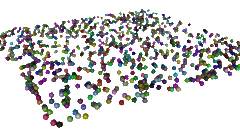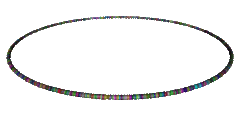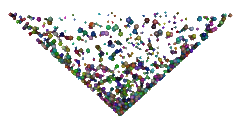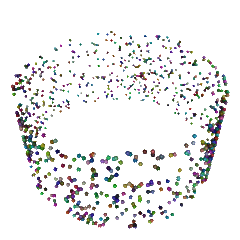### RiPoints on a disk

`#include <stdlib.h>`

 Another tutorial, random points on a sphere covers material that is very similiar to this web page. It is recommended you review that material. Being able to generate random points that are distributed across a disk, or part of a disk, can be helpful when trying to portray a variety of phenomena - spiral arms of hurricanes, galaxies and catherine-wheel firework displays. The first step is to generate a number of vectors in a square - well actually, a cube of zero thickness! ```float xyz; /* generate random points */ for(n = 0; n < 1000; n++) { xyz = randBetween(-1, 1); /* x */ xyz = 0; /* y */ xyz = randBetween(-1, 1); /* z */ /* use xyz in some way, then generate another point */ }``` Next we constrain the random vectors to lie on the rim of a disk?Download the source code Download the RIB file next >>

 USING UNIT VECTORS Instead of considering the random xyz coordinates as defining 3D points consider them as defining the coordinates of random 3D vectors. Therefore, we now have a 1000 vectors of different lengths. By normalizing each vector ie. making them one unit in length, we are in effect distributing them around the perimeter of a disk or circle of radius 1 unit. The xyz coordinate of each position vector can be used to locate a small object, such as a sphere or a (ri)point. A function that performs the normalization is shown opposite.The code on the previous page would be modified to use the normalize() function... ```/* normalize the vectors before using them */ normalize(xyz);``` The next page shows how the points can be randomly distributed to form a disk. ```void normalize(float pnt) { float length = sqrt(pnt * pnt + pnt * pnt + pnt * pnt); pnt /= length; pnt /= length; pnt /= length; }``` << prev next >>

 DISTRIBUTION ACROSS A DISK After normalization the vectors should be scaled by a random value between 0.0 and "radius" - where radius defines the size of a disk. As a consequence the vectors will randomly fill the disk. ```/* normalize the vectors then randomize their positions - ignore the y coordinate */ normalize(xyz); float randomRadius = randBetween(0, radius); xyz *= randomRadius; xyz *= randomRadius;``` If instead of ignoring the y coordinate we do this, ```xyz *= randomRadius; xyz += randomRadius; xyz *= randomRadius;``` ...a cone is formed because the y coordinate of each vector is proportional to its "radius"! Alternatively, if we randomize the y coordinate without effecting the x and z coordinates ie. ```/* xyz *= randomRadius; */ xyz += randomRadius; /* xyz *= randomRadius; */``` ...a cylinder is formed.<< prev next >>